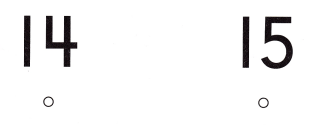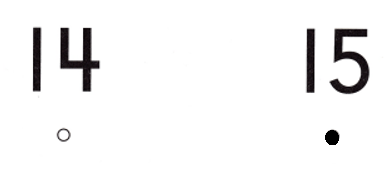# Texas Go Math Kindergarten Lesson 8.8 Answer Key Compare Numbers to 20

Refer to our Texas Go Math Kindergarten Answer Key Pdf to score good marks in the exams. Test yourself by practicing the problems from Texas Go Math Kindergarten Lesson 8.8 Answer Key Compare Numbers to 20.

## Texas Go Math Kindergarten Lesson 8.8 Answer Key Compare Numbers to 20

Unlock the Problem

DIRECTIONS: Alison has more than 15 yellow cubes. Josh has fewer than 17 yellow cubes. Show the cubes, or select an iTool. Compare the sets of cubes. Draw the sets. Tell a friend about your drawing.Explanation:
Alison has more than 15 yellow cubes. Josh has fewer than 17 yellow cubes.
Number of yellow cubes Alison has = 15 + 1 = 16 or Sixteen.
Number of yellow cubes Josh has = 17 – 1 = 16 or Sixteen.

Try Another Problem

DIRECTIONS: 1. Kaelin has 18 apples. Chase has two fewer apples than Kaelin. Use cubes, or select an iTool, to model the sets of apples. Compare the sets. Which set is larger? Draw the sets. Write how many in each set. Circle the greater number. Tell a friend how you compared the numbers.

Question 1.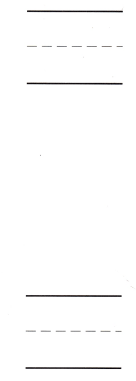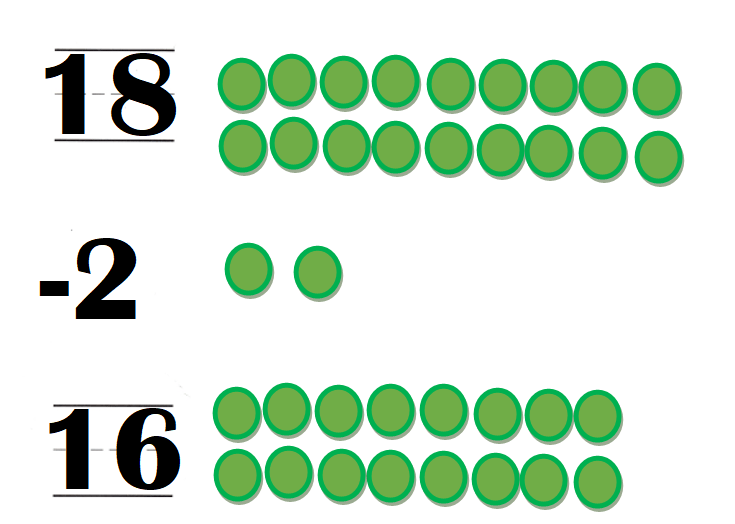Explanation:
Number of apples Kaelin has = 18.
Chase has two fewer apples than Kaelin.
=> Number of apples Chase has  = Number of apples Kaelin has – 2
= 18 – 2
= 16 or Sixteen.

Share and Show

DIRECTIONS: 2. Skyler has 19 oranges. Taylor has two fewer oranges than Skyler. Use cubes, or select an iTool, to model the sets of oranges. Compare the sets. Which set is smaller? Draw the sets. Write how many in each set. Circle the number that is less. Tell a friend how you compared the numbers.

Question 2.Explanation:
Number of oranges Skyler has = 19.
Taylor has two fewer oranges than Skyler.
=> Number of oranges Taylor has = Number of oranges Skyler has  – 2
= 19 – 2
= 17 or Seventeen.

HOME ACTIVITY • Have your child count two sets of objects in your home, and write how many are in each set. Then have him or her circle the greater number. Repeat with sets of different numbers.Explanation:
My kid took green counters of number 19 and 17.
He said 19 is greater than 17 and circled it.

DIRECTIONS: Choose the correct answer. 3. How many balloons are there? 4. How many blocks are there? 5. Which number is greater?

Question 3.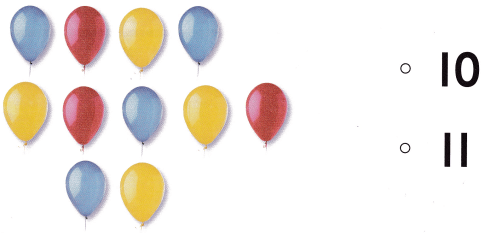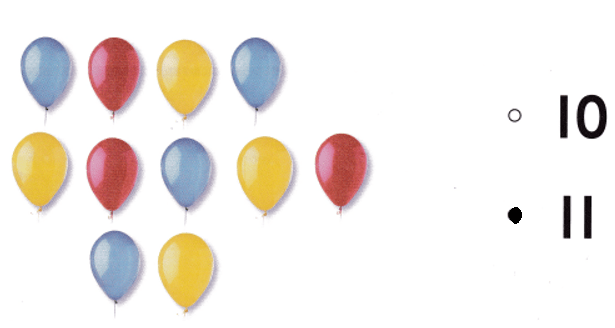Explanation:
Number of balloons = 11 or Eleven.

Question 4.Explanation:
Number of squares = 16 or Sixteen.

Question 5.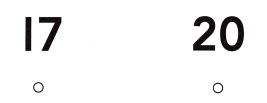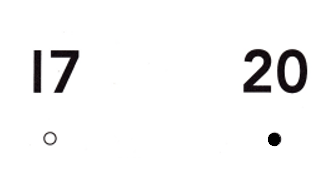Explanation:
Number 20 is greater than number 17.
=> 20 > 17.

### Texas Go Math Kindergarten Lesson 8.8 Homework and Practice Answer Key

DIRECTIONS: 1. Pat has 20 crayons. Alan has two fewer crayons than Pat. Draw the sets of crayons, or select an iTool to show the sets. Compare the sets. Which set is larger? Write how many in each set. Circle the greater number. Tell a friend how you compared the numbers.

Question 1.Explanation:
Number of crayons Pat has = 20.
Alan has two fewer crayons than Pat.
=> Number of crayons Alan has = Number of crayons Pat has -2
= 20 – 2
= 18 or Eighteen.

DIRECTIONS: Choose the correct answer. 2. There are 13 pumpkins. What number is one more than 13? 3. There are 17 ears of corn. What number is one less than 17? 4. Which number greater?

Lesson Check

Question 2.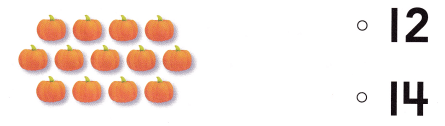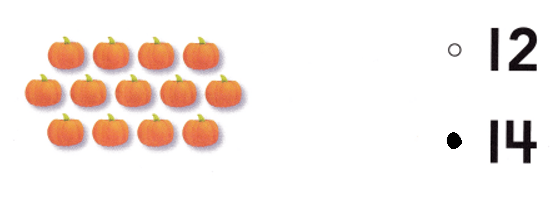Explanation:
Number of pumpkins = 13.
One more than 13 = 13 + 1 = 14 or Fourteen.

Question 3.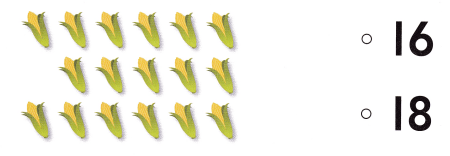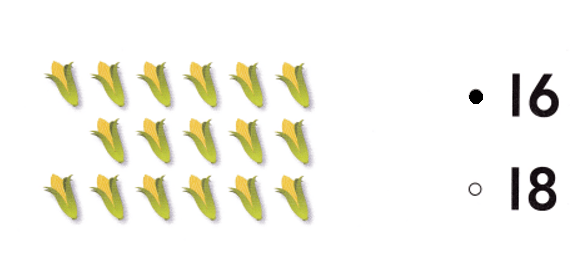Explanation:
Number of  ears of corn = 17.
One less than 17 = 17 – 1 = 16 or Sixteen.

Question 4.